Check Full Chapter Explained - Continuity and Differentiability - https://you.tube/Chapter-5-Class-12-Continuity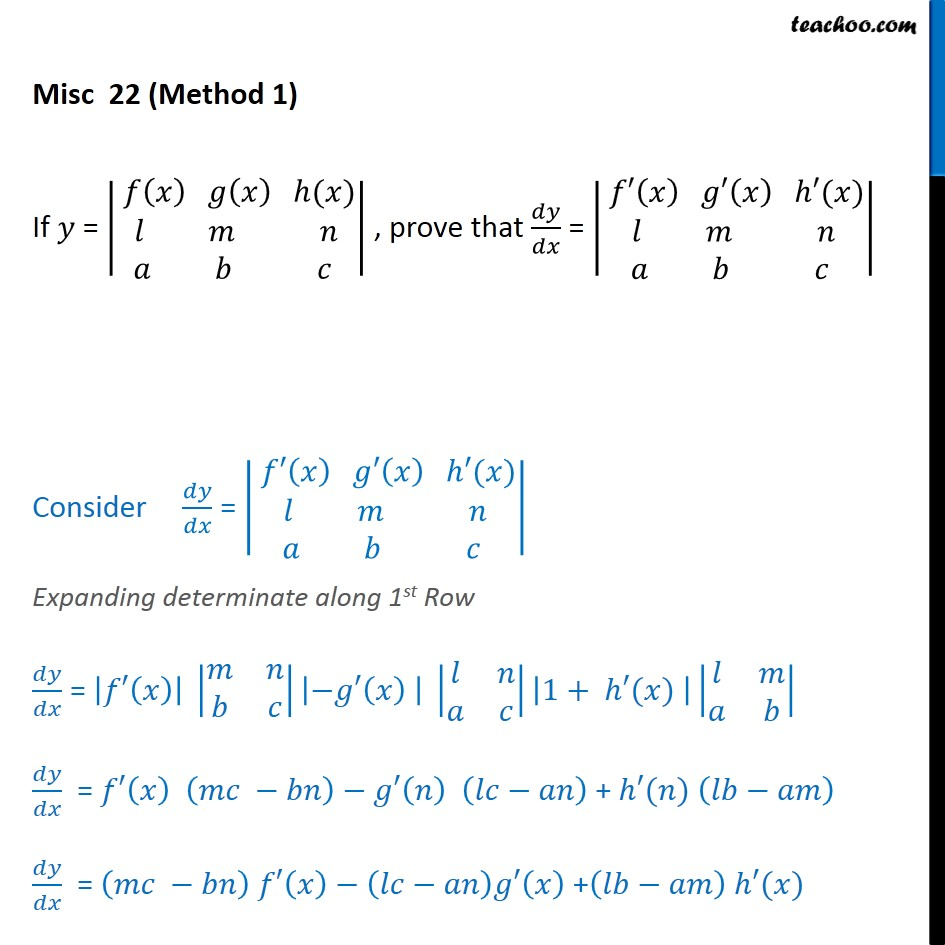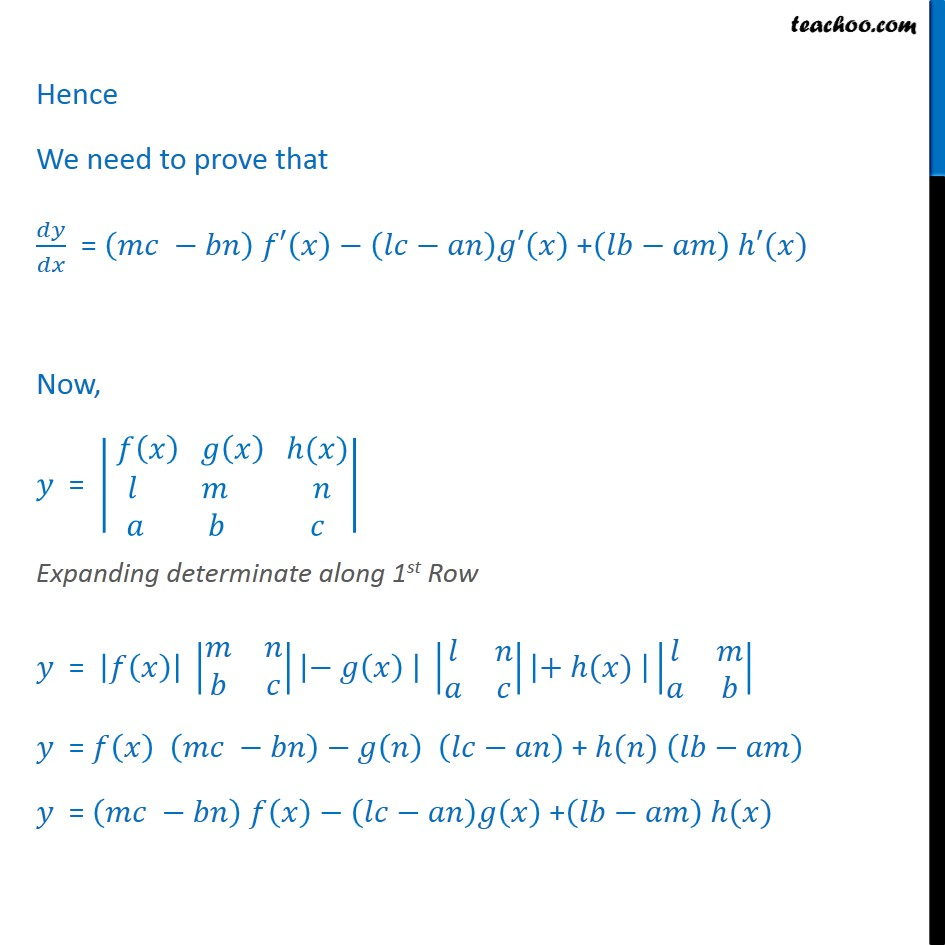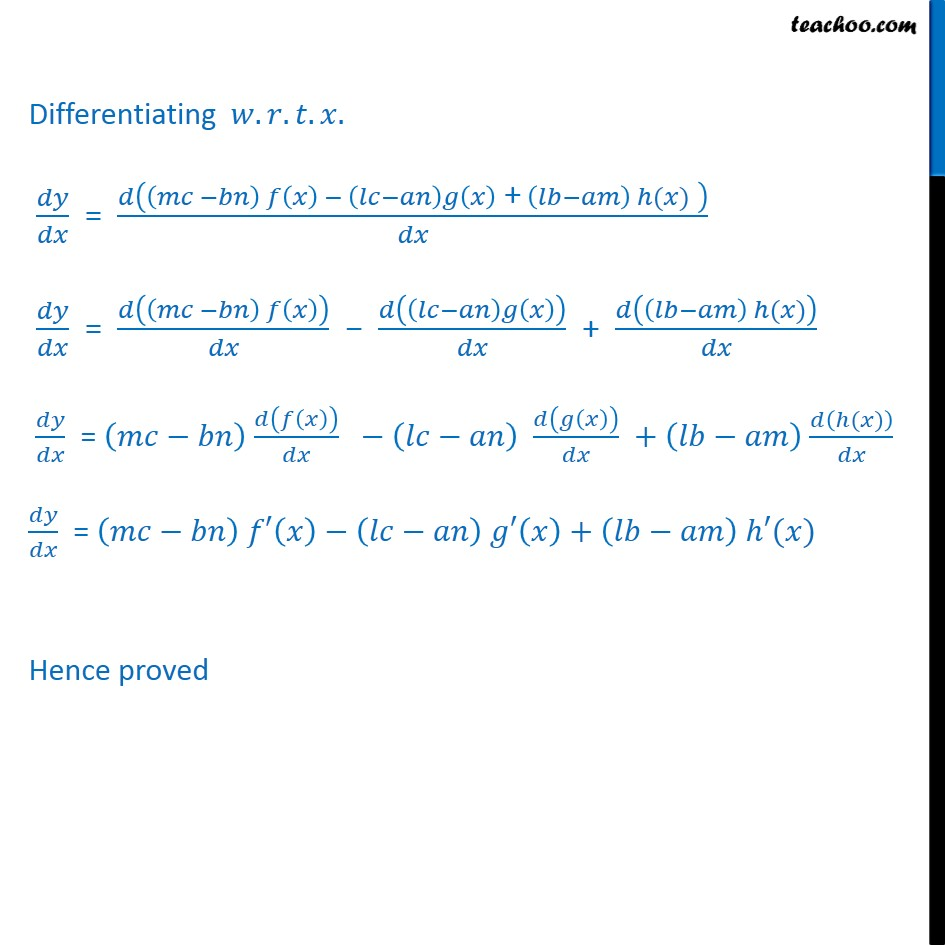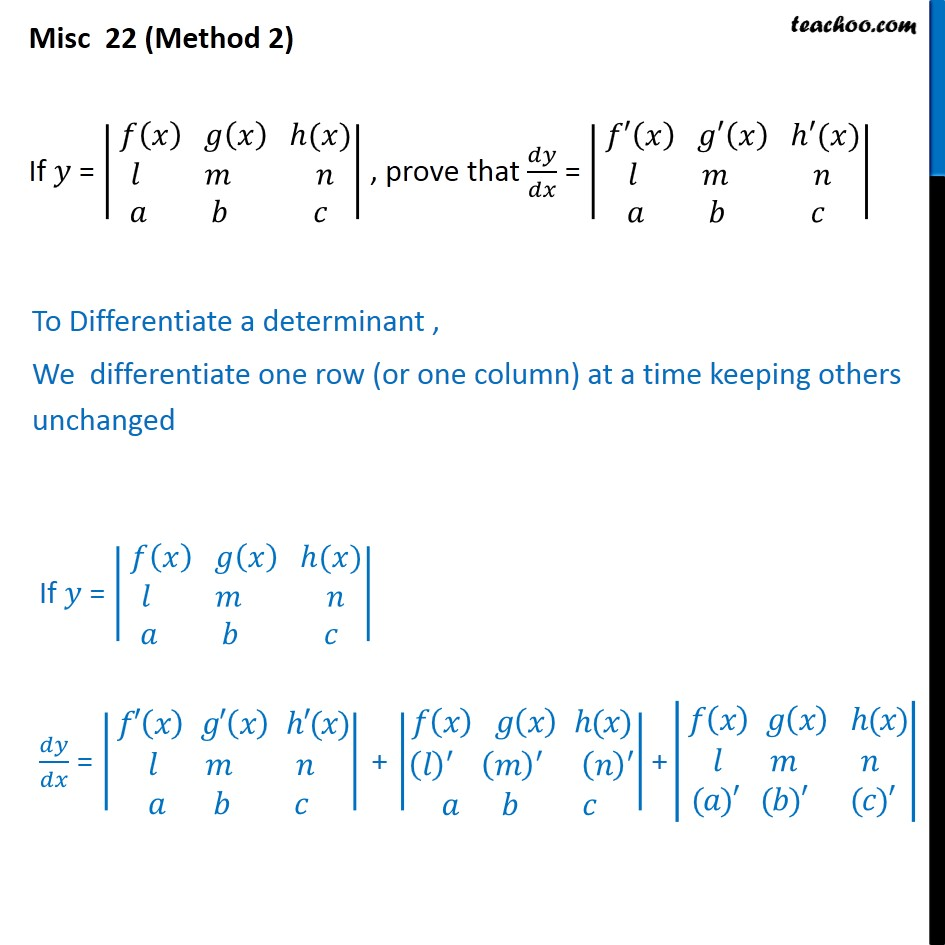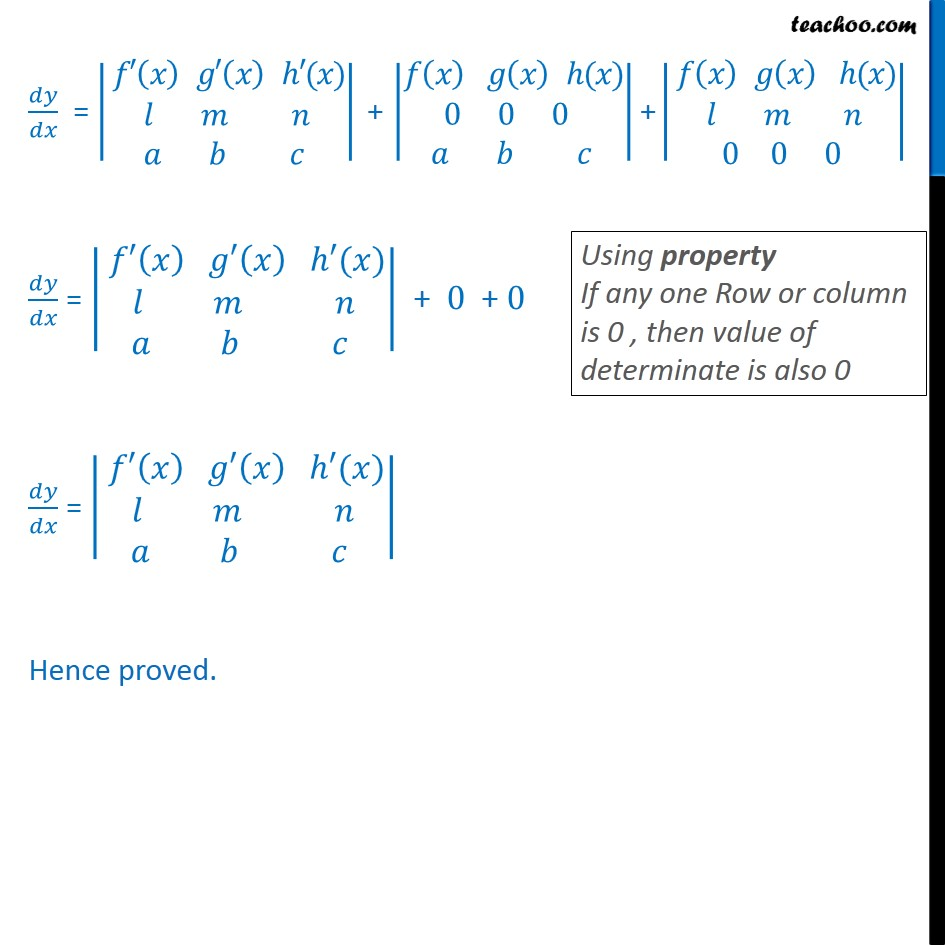1. Chapter 5 Class 12 Continuity and Differentiability
2. Concept wise
3. Proofs

Transcript

Misc 22 (Method 1) If 𝑦 = 𝑓 𝑥﷯ 𝑔 𝑥﷯ ℎ(𝑥)﷮𝑙 𝑚 𝑛﷮𝑎 𝑏 𝑐 ﷯﷯ , prove that 𝑑𝑦﷮𝑑𝑥﷯ = 𝑓′ 𝑥﷯ 𝑔′ 𝑥﷯ ℎ′(𝑥)﷮𝑙 𝑚 𝑛﷮𝑎 𝑏 𝑐 ﷯﷯ Consider 𝑑𝑦﷮𝑑𝑥﷯ = 𝑓′ 𝑥﷯ 𝑔′ 𝑥﷯ ℎ′(𝑥)﷮𝑙 𝑚 𝑛﷮𝑎 𝑏 𝑐 ﷯﷯ Expanding determinate along 1st Row 𝑑𝑦﷮𝑑𝑥﷯ = 𝑓′ 𝑥﷯﷯ 𝑚﷮𝑛﷮𝑏﷮𝑐﷯﷯ −𝑔′ 𝑥﷯ ﷯ 𝑙﷮𝑛﷮𝑎﷮𝑐﷯﷯ 1+ ℎ′(𝑥) ﷯ 𝑙﷮𝑚﷮𝑎﷮𝑏﷯﷯ 𝑑𝑦﷮𝑑𝑥﷯ = 𝑓′ 𝑥﷯ 𝑚𝑐 −𝑏𝑛﷯−𝑔′ 𝑛﷯ 𝑙𝑐−𝑎𝑛﷯ + ℎ′(𝑛) 𝑙𝑏−𝑎𝑚﷯ 𝑑𝑦﷮𝑑𝑥﷯ = 𝑚𝑐 −𝑏𝑛﷯ 𝑓′ 𝑥﷯− 𝑙𝑐−𝑎𝑛﷯𝑔′ 𝑥﷯ + 𝑙𝑏−𝑎𝑚﷯ ℎ′(𝑥) Hence We need to prove that 𝑑𝑦﷮𝑑𝑥﷯ = 𝑚𝑐 −𝑏𝑛﷯ 𝑓′ 𝑥﷯− 𝑙𝑐−𝑎𝑛﷯𝑔′ 𝑥﷯ + 𝑙𝑏−𝑎𝑚﷯ ℎ′(𝑥) Now, 𝑦 = 𝑓 𝑥﷯ 𝑔 𝑥﷯ ℎ(𝑥)﷮𝑙 𝑚 𝑛﷮𝑎 𝑏 𝑐 ﷯﷯ Expanding determinate along 1st Row 𝑦 = 𝑓 𝑥﷯﷯ 𝑚﷮𝑛﷮𝑏﷮𝑐﷯﷯ − 𝑔 𝑥﷯ ﷯ 𝑙﷮𝑛﷮𝑎﷮𝑐﷯﷯ + ℎ(𝑥) ﷯ 𝑙﷮𝑚﷮𝑎﷮𝑏﷯﷯ 𝑦 = 𝑓 𝑥﷯ 𝑚𝑐 −𝑏𝑛﷯−𝑔 𝑛﷯ 𝑙𝑐−𝑎𝑛﷯ + ℎ(𝑛) 𝑙𝑏−𝑎𝑚﷯ 𝑦 = 𝑚𝑐 −𝑏𝑛﷯ 𝑓 𝑥﷯− 𝑙𝑐−𝑎𝑛﷯𝑔 𝑥﷯ + 𝑙𝑏−𝑎𝑚﷯ ℎ(𝑥) Differentiating 𝑤.𝑟.𝑡.𝑥. 𝑑𝑦﷮𝑑𝑥﷯ = 𝑑 𝑚𝑐 −𝑏𝑛﷯ 𝑓 𝑥﷯ − 𝑙𝑐−𝑎𝑛﷯𝑔 𝑥﷯ + 𝑙𝑏−𝑎𝑚﷯ ℎ(𝑥) ﷯﷮𝑑𝑥﷯ 𝑑𝑦﷮𝑑𝑥﷯ = 𝑑 𝑚𝑐 −𝑏𝑛﷯ 𝑓 𝑥﷯﷯﷮𝑑𝑥﷯ − 𝑑 𝑙𝑐−𝑎𝑛﷯𝑔 𝑥﷯﷯﷮𝑑𝑥﷯ + 𝑑 𝑙𝑏−𝑎𝑚﷯ ℎ(𝑥)﷯﷮𝑑𝑥﷯ 𝑑𝑦﷮𝑑𝑥﷯ = 𝑚𝑐−𝑏𝑛﷯ 𝑑 𝑓 𝑥﷯﷯﷮𝑑𝑥﷯ − 𝑙𝑐−𝑎𝑛﷯ 𝑑 𝑔 𝑥﷯﷯﷮𝑑𝑥﷯ + 𝑙𝑏−𝑎𝑚﷯ 𝑑 ℎ(𝑥)﷯﷮𝑑𝑥﷯ 𝑑𝑦﷮𝑑𝑥﷯ = 𝑚𝑐−𝑏𝑛﷯ 𝑓′ 𝑥﷯− 𝑙𝑐−𝑎𝑛﷯ 𝑔′ 𝑥﷯ + 𝑙𝑏−𝑎𝑚﷯ ℎ′(𝑥) Hence proved Misc 22 (Method 2) If 𝑦 = 𝑓 𝑥﷯ 𝑔 𝑥﷯ ℎ(𝑥)﷮𝑙 𝑚 𝑛﷮𝑎 𝑏 𝑐 ﷯﷯ , prove that 𝑑𝑦﷮𝑑𝑥﷯ = 𝑓′ 𝑥﷯ 𝑔′ 𝑥﷯ ℎ′(𝑥)﷮𝑙 𝑚 𝑛﷮𝑎 𝑏 𝑐 ﷯﷯ To Differentiate a determinant , We differentiate one row (or one column) at a time keeping others unchanged If 𝑦 = 𝑓 𝑥﷯ 𝑔 𝑥﷯ ℎ(𝑥)﷮𝑙 𝑚 𝑛﷮𝑎 𝑏 𝑐 ﷯﷯ 𝑑𝑦﷮𝑑𝑥﷯ = 𝑓′ 𝑥﷯ 𝑔′ 𝑥﷯ ℎ′(𝑥)﷮𝑙 𝑚 𝑛﷮𝑎 𝑏 𝑐 ﷯﷯ + 𝑓 𝑥﷯ 𝑔 𝑥﷯ ℎ(𝑥)﷮ 𝑙﷯﷮′﷯ 𝑚﷯﷮′﷯ 𝑛﷯﷮′﷯﷮𝑎 𝑏 𝑐 ﷯﷯ + 𝑓 𝑥﷯ 𝑔 𝑥﷯ ℎ(𝑥)﷮𝑙 𝑚 𝑛﷮(𝑎)′ (𝑏)′ (𝑐)′ ﷯﷯ 𝑑𝑦﷮𝑑𝑥﷯ = 𝑓′ 𝑥﷯ 𝑔′ 𝑥﷯ ℎ′(𝑥)﷮𝑙 𝑚 𝑛﷮𝑎 𝑏 𝑐 ﷯﷯ + 𝑓 𝑥﷯ 𝑔 𝑥﷯ ℎ(𝑥)﷮0 0 0 ﷮𝑎 𝑏 𝑐 ﷯﷯ + 𝑓 𝑥﷯ 𝑔 𝑥﷯ ℎ(𝑥)﷮𝑙 𝑚 𝑛﷮0 0 0 ﷯﷯ 𝑑𝑦﷮𝑑𝑥﷯ = 𝑓′ 𝑥﷯ 𝑔′ 𝑥﷯ ℎ′(𝑥)﷮𝑙 𝑚 𝑛﷮𝑎 𝑏 𝑐 ﷯﷯ + 0 + 0 𝑑𝑦﷮𝑑𝑥﷯ = 𝑓′ 𝑥﷯ 𝑔′ 𝑥﷯ ℎ′(𝑥)﷮𝑙 𝑚 𝑛﷮𝑎 𝑏 𝑐 ﷯﷯ Hence proved.

Proofs